This mathematics ClipArt gallery offers 127 images that can be used to demonstrate various geometric theorems and proofs.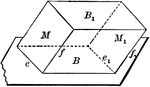### Equal and Parallel Opposite Faces of a Parallelopiped

Diagram used to prove the theorem: "The opposite faces of a parallelopiped are equal and parallel."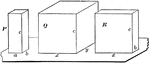### Relationship Between 2 Parallelopipeds With Equal Altitudes

Diagram used to prove the theorem: "The rectangular parallelopipeds which have two dimensions in common…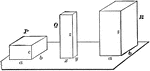### Relationship Between Dimensions of Parallelopipeds

Diagram used to prove the theorem: "The rectangular parallelopipeds are to each other as the product…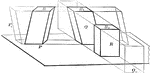### Volume of Parallelopiped

Diagram used to prove the theorem: "The volume of a any parallelopiped is equal to the product of its…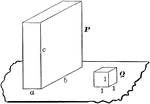### Volume of Rectangular Parallelopiped

Diagram used to prove the theorem: "The volume of a rectangular parallelopiped is equal to the product…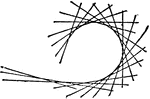### Plane Curve

A line moves in a plane and it therefore envelopes a plane curve.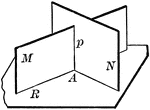### 2 Intersecting Planes Perpendicular To A Third Plane

"If two intersecting planes are each perpendicular to a third plane, their intersection is also perpendicular…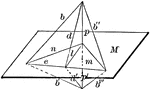### Line Perpendicular To Plane

"If a straight line is perpendicular to each of two straight lines at their point of intersection it…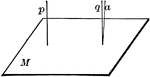### Lines Perpendicular To A Plane

"If one of two parallels is perpendicular to a plane, the other is also."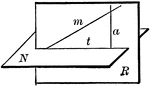### Plane Passed Perpendicular To A Given Plane

"Through a given line oblique to a plane, one, and only one plane, can be passed perpendicular to the…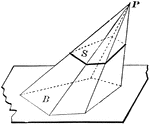### Pyramid Cut By Plane

Diagram used to prove the theorem: "If a pyramid is cut by a plane parallel to the base, the edges are…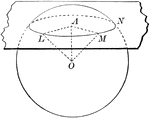### Sphere Cut By Plane

Diagram used to prove the theorem: "Every section of a sphere by a lane is a circle."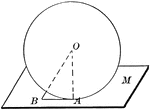### Sphere Tangent to Plane

Diagram used to prove the theorem: "A plane perpendicular to a radius at its extremity is tangent to…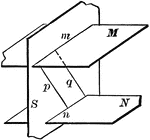### 3 Intersecting Planes

Illustration of three intersecting planes. "The intersections of two parallel planes by a third plane…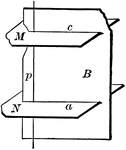### 3 Intersecting Planes

Illustration of three intersecting planes. "The straight line perpendicular to one of two parallel lanes…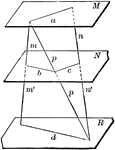### 3 Parallel Planes

"If two straight lines are cut by three parallel planes, the corresponding segments are proportional."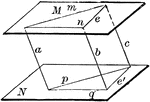### Angles In Parallel Planes

"If two angles not in the same plane have their sides respectively parallel and lying in the same direction,…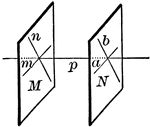### Parallel Planes

"If two intersecting lines are each parallel to a given plane, the plane of these lines is parallel…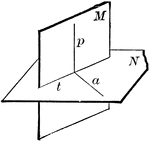### Perpendicular Planes

"If two planes are perpendicular to each other, a line in one of them perpendicular to the intersection…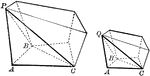### Two Similar Polyhedrons

Diagram used to prove the theorem: "Two similar polyhedrons may be decomposed into the same number of…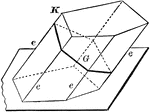### Lateral Area of A Prism

Diagram used to prove the theorem: "The lateral area of a prism is equal to the product of a lateral…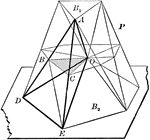### Volume of Prismatoid

Diagram used to prove the theorem: "The volume of a prismatoid is equal to the product of one-sixth…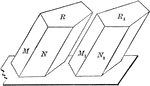### Two Equal Prisms

Diagram used to prove the theorem: "Two prisms are equal when the three faces about a trihedral of one…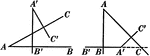### Angles With Perpendicular Sides Are Equal or Supplementary Proof

Illustration used to prove "Two angles whose sides are perpendicular each to each are either equal or…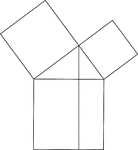### Euclid's Pythagorean Theorem Proof

Illustration used to prove the Pythagorean Theorem, according to Euclid. A perpendicular is drawn from…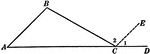### Exterior Angle Proof

Illustration used to prove "An exterior angle of a triangle is equal to the sum of the two remote interior…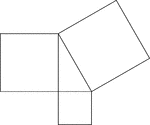### Geometric Pythagorean Theorem Proof

Illustration that can be used to prove the Pythagorean Theorem, the sum of the squares of the legs is…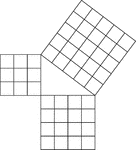### Geometric Pythagorean Theorem Proof

Illustration that can be used to prove the Pythagorean Theorem, the sum of the squares of the legs is…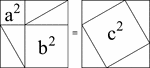### Pythagorean Theorem Proof by Rearrangement

A visual illustration used to prove the Pythagorean Theorem by rearrangement. When the 4 identical triangles…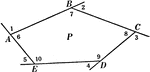### Sum of Exterior Angles of a Polygon Proof

Illustration used to prove "If the sides of any polygon are prolonged in succession one way, no two…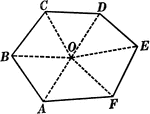### Sum of Interior Angles of a Polygon Proof

Illustration used to prove "The sum of all the angles of any polygon is twice as many right angles as…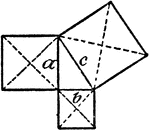### Model Of Pythagorean Theorem

An illustration showing a model that illustrates the Pythagorean Theorem: a² + b² = c².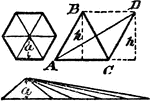### Area Of Regular Polygon Proof

An illustration showing that the area of a regular polygon is equal to the area of a triangle whose…### Relationships In A Right Triangle

Illustration used to show "If one acute angle of a right triangle is double the other, the hypotenuse…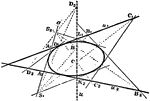### Second Order Curve

Five points are given, of which not three are in a line, a curve of second order may be drawn through…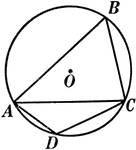### Right Angles Inscribed in Semicircle Proof

Illustration of a circle used to prove "Any angle inscribed in a semicircle is a right angle."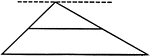### Line Bisecting Side of a Triangle

Illustration used to prove "The line bisecting one side of a triangle and parallel to another side bisects…### Circumference of a Circle of a Sphere

Diagram used to prove the theorem: "All points in the circumference of a circle of a sphere are equally…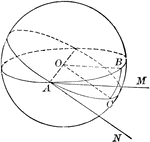### Measure of a Spherical Angle

Diagram used to prove the theorem: "A spherical angle is measured by the arc of a great circle described…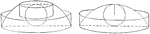### Volume of Spherical Segment

Diagram used to prove the theorem: "The volume of a spherical segment is equal to the sum of two cylinders…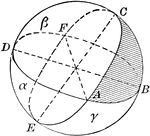### Area of Spherical Triangle

Diagram used to prove the theorem: "The area of a spherical triangle, expressed in spherical degrees,…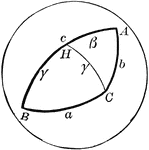### Side Comparison of Spherical Triangle

Diagram used to prove the theorem: "In a spherical triangle, the greater side is opposite the greater…### Perpendicular Between 2 Straight Lines

Diagram used to prove the theorem: "Between two straight lines not in the same plane only one perpendicular…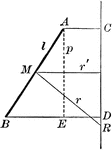### Area of Surface Generated by a Straight Line

Diagram used to prove the theorem: "The area of the surface generated by a straight line revolving about…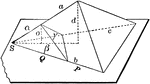### Two Proportional Tetrahedrons

Diagram used to prove the theorem: "Two tetrahedrons having a trihedral angle in each equal, are to…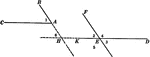### 2 Angles With Parallel Sides Theorem

Illustration used to prove the theorem, "Two angles whose sides are parallel, each to each, are either…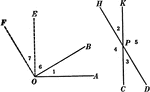### 2 Angles With Perpendicular Sides Theorem

Illustration used to prove the theorem, "Two angles whose sides are perpendicular, each to each, are…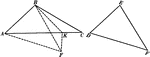### 2 Triangles Theorem

Illustration used to prove that "If two triangles have two sides of one equal respectively to two sides…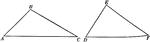### 2 Triangles Theorem

Illustration used to prove that "If two triangles have two sides of one equal respectively to two sides…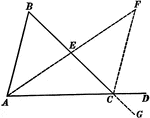### Exterior Angle of Triangle Theorem

Illustration used to prove that "If one side of a triangle is prolonged, the exterior angle formed is…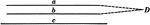### Parallel Lines Theorem

Illustration used to prove that "If two straight lines are parallel to a third straight line, they are…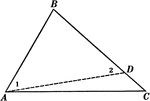### Sides of Triangle Theorem

Illustration used to prove that "If two sides of a triangle are unequal, the angle opposite the greater…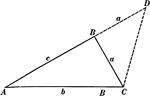### Sides of Triangle Theorem

Illustration used to prove that "The sum of any two sides of a triangle is greater than the third side."### Sum Of Angles In Triangle Theorem

Illustration used to prove the theorem, "The sum of the angles of any triangle is two right angles."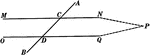### Parallel Lines Cut By A Transversal Theorem

Illustration used to prove the theorem, "If two straight lines are cut by a transversal making a pair…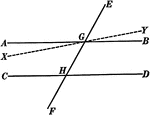### Parallel Lines Cut By A Transversal Theorem

Illustration used to prove the theorem, "If two parallel lines are cut by a transversal, the alternate…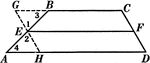### Line Bisecting Trapezoid Sides

Illustration used to prove the theorem "The line bisecting one of the non parallel sides of a trapezoid…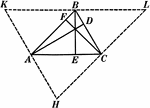### Altitudes In A Triangle

Illustration used to show "The altitudes of a triangle are concurrent."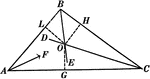### Angle Bisectors In A Triangle

Illustration used to prove "The bisectors of the angles of a triangle are concurrent in a point which…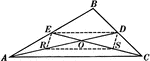### Medians In A Triangle

Illustration used to show "Any two medians of a triangle intersect each other in a trisection point…